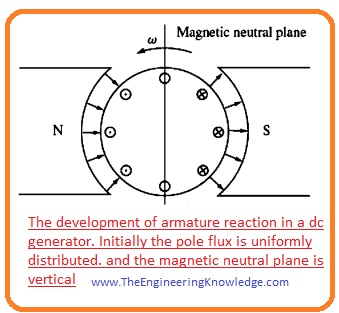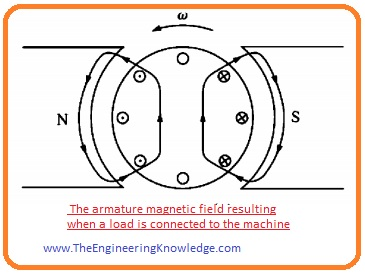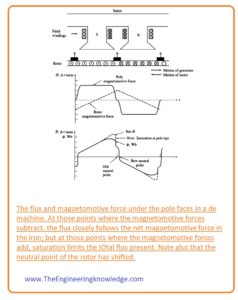Hello, friends, I hope all of you are having fun in your life. In today’s tutorial, we will discuss the Commutation Problems in DC MachinesIn dc machines the voltage produce is ac voltage like output voltage of ac generators such as induction generator and synchronous generator. For a generation of these voltages, we have discussed the rotation of a simple loop in a field. For conversion of ac voltage produce in the dc machines half of the ring is connected this split ring is known as commutators and the process of AC to DC conversion is called commutation.

During this commutation, there are some problems occurs that effect the ac to dc conversion process. In today’s post, we will have a look at these issues that disturb the commutation and also discuss some solutions to these problems. So let’s get started with Problems with Commutation in DC Machines.

#### Commutation Problems in DC Machines

• There are 2 main issues that disturb the commutation process. We will discuss these two with details and solutions of these problems.
• So friends let’s first discuss Armature’s reaction.
• If we connect input supply at the stator of dc machine and also rotates the rotor of a machine with the exterior prime mover, during rotation rotor will link with the flux of stator field that will induce a voltage in the rotor.
• The voltage will be AC and covert into dc by the commutator attached to the rotor. Now if we connect some load with the rotor of a machine it will use some amount of current that will pass through the armature or rotor windings.
• Due to this current, there will be another magnetic field will produce that will affect the field of the stator.
• This disturbance of the armature field to the original field of stator is known as armature reaction.
• There are two main issues occurs due to this armature reaction.
• Neutral-Plane Shift
• Flux WeakeningNeutral plane shift

• The ist issue caused by the armature reaction is a neutral plane shift, in dc machine a position where the rotation direction of the rotor is parallel to field lines this position is known as a magnetic neutral plane.
• In this plane voltage production in armature windings is zero.
• For an understanding of the neutral plane shift problem, we discuss the given below the figure, which has 2 pole dc machines.• You can see that flux is uniform over the complete machine. The voltage induced in the winding under the North Pole is out of page and voltage to induce in rotor windings under the South Pole has direction into a page.
• You can see that in an above-given figure the direction of the neutral plane is at ninety degrees. It is when there is no load is linked with the machine.
• Let’s assume that we have connected a load with this machine it behaves like a generator. Current will flow in outward direction form positive output terminal, thus current direction is out of the page for windings under the effect of north poles and into a page for windings effect of south poles.
• Due to this current, there will be field produce in the windings of the rotor it is shown in this given figure.• This field of a rotor will interact with the field generated by the stator of the generator. At some points, these two fields will add up with each other and some points will cancel each other.
• The final effect of these two fields is shown in this given figure.• You can see that the point where the voltage produce is 0, where the neutral plane shift has occurred.
• As we are working on dc generator you can observe that the neutral plane shift is in the direction in which the generator is rotating.
• But if it is the dc motor then the neutral plane shift will be opposite to the direction of rotation.
• In simple words, the plane shift for a generator is in direction of rotation and for a motor is in the opposite direction of rotation.
• The plane shift relies on the quantity of current drawn from the rotor and load connected.

So what’s the big deal about the neutral-plane shift?

• The commutator necessity short-circuited commutator segments at the instant when the voltage crosswise them is 0.
• If the brushes are fixed to short-out conductors in the perpendicular plane, then the voltage among segments is certainly 0 till the load is attached.
• When a load is attached, the neutral plane shifts and the brushes short-circuited commutator segments with a specific value of voltage crossways them.
• The consequence is a current now flowing among the short out segments and huge sparks at the brushes when the current is disturbed as the brush left a segment. The final outcome is arcing and sparking at the brushes.
• It is a thoughtful problematic, as it leads to severely decreases brush Life, pitting of the commutator segments, and highly enhance repairing charges.
• Note that this issue cannot be removed even by insertion the brushes over the full-load neutral plane since sparking will occur at no-load condition.

Flux weakening Armature Reaction

• Mostly machine work at flux near the saturation point. So at the point where the mmf of rotor adds up with the MMF of poles at stator, there is less increment in total flux.
• But at a point where mmf rotor subtracted from the mmf poles then larger decrement occurs in flux value.
• The final outcome is that the net value of flux over a complete pole will be lessened.You can see this in a given figure.• The weakening of flux is not good for both motor and generator. In generators, the output voltage of the generator decreases.
• But in the case of the motor, this factor causes serious problems. With the decrement of a flux rotation speed of motor also decreases.
• Speed increment also enhances the load connected with the motor that causes a lessening of flux.
• It is likely in some shunt dc motors to approaches a running state as a consequence of flux decrement (weakening), where the speed of the motor increasing till the machine is detached with input supply or until it damage.

#### L di/dt Voltages

• The 2nd issue that occurs during commutation is L di/dt that occurs on the commutator is also known as an inductive kick.
• To an understanding of this issue lets discuss this given figure. You can see commutator segments are attached with one another in a sequence and winding conductor is linked with them.• Let’s suppose that current passing through the carbon brush is four hundred amperes, the current through every path is two hundred amperes.
• Note that when the commutator is short-circuited, the current passing through the commutator will be reversed.
• Here the question arises.

How fast must this reverse occur?

• Let’s suppose that the machine is rotating at the eight hundred revolutions per minute and have fifty commutators segments every commutator segment rotates under a brush and change its position in (t = 0.00 15 seconds).

di/dt = 400A/0.0015s= 266,667 A/s

• With a small value of inductance, a very high value of Ldi/dt will be produced at the short-circuited commutator segments.
• This higher voltage obviously sources flashing at the brushes of the machine, resultant in the similar arcing problems that the neutral-plane shift causes.

So friends that is the detailed post about Problems with Commutation in DC Machines I have mentioned problems and their solutions. If you still have any query ask in comments. See you in the next tutorial. Thanks for reading.